### Step

The geometry of a step is the inverse of the drop geometry. Although a step is really a discontinuity, a small fictitious length an a slope have to be assigned. For the slope one can take the mean values of the slopes of the adjacent channels. The following constants have to be specified on the line beneath the *FLUID SECTION,TYPE=CHANNEL STEP card:

• the width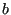• the slope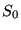(if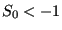zero is taken)
• the length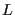(if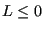the length is calculated from the coordinates of the end nodes belonging to the element)
• the step size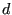Example files: channel10, channel12.